Category: groups

Thanks for clicking through… I guess.

If nothing else, it shows that just as much as the stock market is fueled by greed, mathematical reasearch is driven by frustration (or the pleasure gained from knowing others to be frustrated).

I did spend the better part of the day doing a lengthy, if not laborious, calculation, I’ve been postponing for several years now. Partly, because I didn’t know how to start performing it (though the basic strategy was clear), partly, because I knew beforehand the final answer would probably offer me no further insight.

Still, it gives the final answer to a problem that may be of interest to anyone vaguely interested in Moonshine :

What does the Monster see of the modular group?

I know at least two of you, occasionally reading this blog, understand what I was trying to do and may now wonder how to repeat the straightforward calculation. Well the simple answer is : Google for the number 97239461142009186000 and, no doubt, you will be able to do the computation overnight.

One word of advice : don’t! Get some sleep instead, or make love to your partner, because all you’ll get is a quiver on nine vertices (which is pretty good for the Monster) but having an horrible amount of loops and arrows…

If someone wants the details on all of this, just ask. But, if you really want to get me exited : find a moonshine reason for one of the following two numbers :

$791616381395932409265430144165764500492= 2^2 * 11 * 293 * 61403690769153925633371869699485301$

(the dimension of the monster-singularity upto smooth equivalence), or,

$1575918800531316887592467826675348205163= 523 * 1655089391 * 15982020053213 * 113914503502907$

(the dimension of the moduli space).Referring to the triple of exceptional Galois groups $L_2(5),L_2(7),L_2(11)$ and its connection to the Platonic solids I wrote : “It sure seems that surprises often come in triples…”. Briefly I considered replacing triples by trinities, but then, I didnt want to sound too mystic…

David Corfield of the n-category cafe and a dialogue on infinity (and perhaps other blogs I’m unaware of) pointed me to the paper Symplectization, complexification and mathematical trinities by Vladimir I. Arnold. (Update : here is a PDF-conversion of the paper)

The paper is a write-up of the second in a series of three lectures Arnold gave in june 1997 at the meeting in the Fields Institute dedicated to his 60th birthday. The goal of that lecture was to explain some mathematical dreams he had.

The next dream I want to present is an even more fantastic set of theorems and conjectures. Here I also have no theory and actually the ideas form a kind of religion rather than mathematics.
The key observation is that in mathematics one encounters many trinities. I shall present a list of examples. The main dream (or conjecture) is that all these trinities are united by some rectangular “commutative diagrams”.
I mean the existence of some “functorial” constructions connecting different trinities. The knowledge of the existence of these diagrams provides some new conjectures which might turn to be true theorems.

Follows a list of 12 trinities, many taken from Arnold’s field of expertise being differential geometry. I’ll restrict to the more algebraically inclined ones.

1 : “The first trinity everyone knows is”where $\mathbb{H}$ are the Hamiltonian quaternions. The trinity on the left may be natural to differential geometers who see real and complex and hyper-Kaehler manifolds as distinct but related beasts, but I’m willing to bet that most algebraists would settle for the trinity on the right where $\mathbb{O}$ are the octonions.

2 : The next trinity is that of the exceptional Lie algebras E6, E7 and E8.with corresponding Dynkin-Coxeter diagramsArnold has this to say about the apparent ubiquity of Dynkin diagrams in mathematics.

Manin told me once that the reason why we always encounter this list in many different mathematical classifications is its presence in the hardware of our brain (which is thus unable to discover a more complicated scheme).
I still hope there exists a better reason that once should be discovered.

Amen to that. I’m quite hopeful human evolution will overcome the limitations of Manin’s brain…

3 : Next comes the Platonic trinity of the tetrahedron, cube and dodecahedronClearly one can argue against this trinity as follows : a tetrahedron is a bunch of triangles such that there are exactly 3 of them meeting in each vertex, a cube is a bunch of squares, again 3 meeting in every vertex, a dodecahedron is a bunch of pentagons 3 meeting in every vertex… and we can continue the pattern. What should be a bunch a hexagons such that in each vertex exactly 3 of them meet? Well, only one possibility : it must be the hexagonal tiling (on the left below). And in normal Euclidian space we cannot have a bunch of septagons such that three of them meet in every vertex, but in hyperbolic geometry this is still possible and leads to the Klein quartic (on the right). Check out this wonderful post by John Baez for more on this.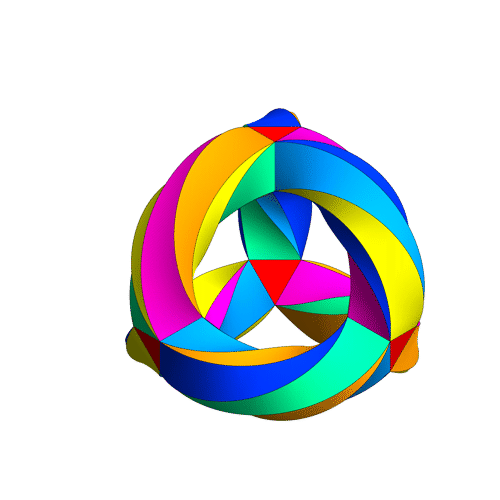4 : The trinity of the rotation symmetry groups of the three Platonicswhere $A_n$ is the alternating group on n letters and $S_n$ is the symmetric group.

Clearly, any rotation of a Platonic solid takes vertices to vertices, edges to edges and faces to faces. For the tetrahedron we can easily see the 4 of the group $A_4$, say the 4 vertices. But what is the 4 of $S_4$ in the case of a cube? Well, a cube has 4 body-diagonals and they are permuted under the rotational symmetries. The most difficult case is to see the $5$ of $A_5$ in the dodecahedron. Well, here’s the solution to this riddle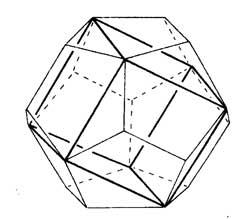there are exactly 5 inscribed cubes in a dodecahedron and they are permuted by the rotations in the same way as $A_5$.

7 : The seventh trinity involves complex polynomials in one variablethe Laurant polynomials and the modular polynomials (that is, rational functions with three poles at 0,1 and $\infty$.

8 : The eight one is another beautyHere ‘numbers’ are the ordinary complex numbers $\mathbb{C}$, the ‘trigonometric numbers’ are the quantum version of those (aka q-numbers) which is a one-parameter deformation and finally, the ‘elliptic numbers’ are a two-dimensional deformation. If you ever encountered a Sklyanin algebra this will sound familiar.

This trinity is based on a paper of Turaev and Frenkel and I must come back to it some time…

The paper has some other nice trinities (such as those among Whitney, Chern and Pontryagin classes) but as I cannot add anything sensible to it, let us include a few more algebraic trinities. The first one attributed by Arnold to John McKay

13 : A trinity parallel to the exceptional Lie algebra one isbetween the 27 straight lines on a cubic surface, the 28 bitangents on a quartic plane curve and the 120 tritangent planes of a canonic sextic curve of genus 4.

14 : The exceptional Galois groupsexplained last time.

15 : The associated curves with these groups as symmetry groups (as in the previous post)where the ? refers to the mysterious genus 70 curve. I’ll check with one of the authors whether there is still an embargo on the content of this paper and if not come back to it in full detail.

16 : The three generations of sporadic groupsDo you have other trinities you’d like to worship?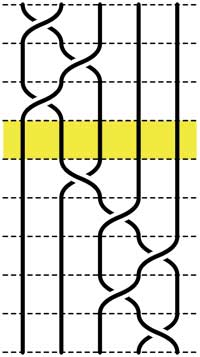Recall that an n-braid consists of n strictly descending elastic strings connecting n inputs at the top (named 1,2,…,n) to n outputs at the bottom (labeled 1,2,…,n) upto isotopy (meaning that we may pull and rearrange the strings in any way possible within 3-dimensional space). We can always change the braid slightly such that we can divide the interval between in- and output in a number of subintervals such that in each of those there is at most one crossing.

n-braids can be multiplied by putting them on top of each other and connecting the outputs of the first braid trivially to the inputs of the second. For example the 5-braid on the left can be written as $B=B_1.B_2$ with $B_1$ the braid on the top 3 subintervals and $B_2$ the braid on the lower 5 subintervals.

In this way (and using our claim that there can be at most 1 crossing in each subinterval) we can write any n-braid as a word in the generators $\sigma_i$ (with $1 \leq i < n$) being the overcrossing between inputs i and i+1. Observe that the undercrossing is then the inverse $\sigma_i^{-1}$. For example, the braid on the left corresponds to the word

$\sigma_1^{-1}.\sigma_2^{-1}.\sigma_1^{-1}.\sigma_2.\sigma_3^{-1}.\sigma_4^{-1}.\sigma_3^{-1}.\sigma_4$

Clearly there are relations among words in the generators. The easiest one we have already used implicitly namely that $\sigma_i.\sigma_i^{-1}$ is the trivial braid. Emil Artin proved in the 1930-ies that all such relations are consequences of two sets of ‘obvious’ relations. The first being commutation relations between crossings when the strings are far enough from each other. That is we have

$\sigma_i . \sigma_j = \sigma_j . \sigma_i$ whenever $|i-j| \geq 2$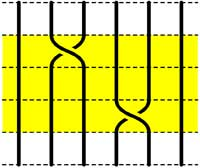=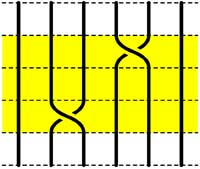The second basic set of relations involves crossings using a common string

$\sigma_i.\sigma_{i+1}.\sigma_i = \sigma_{i+1}.\sigma_i.\sigma_{i+1}$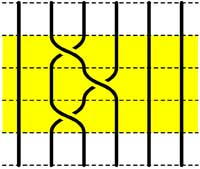=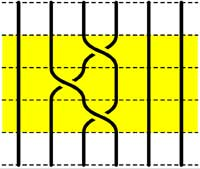Starting with the 5-braid at the top, we can use these relations to reduce it to a simpler form. At each step we have outlined to region where the relations are applied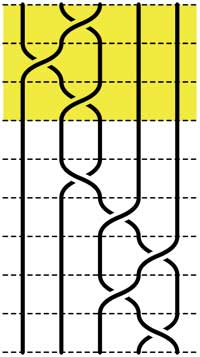=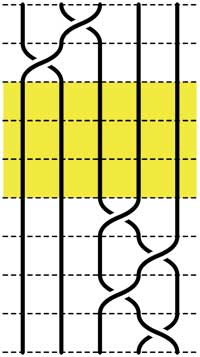=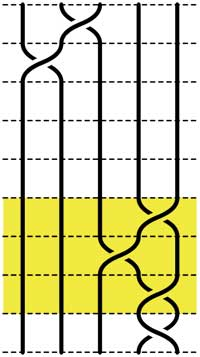=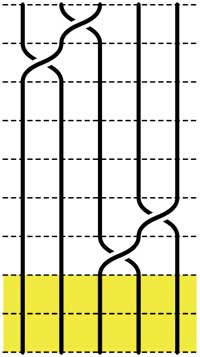These beautiful braid-pictures were produced using the braid-metapost program written by Stijn Symens.

Tracing a string from an input to an output assigns to an n-braid a permutation on n letters. In the above example, the permutation is $~(1,2,4,5,3)$. As this permutation doesn’t change under applying basic reduction, this gives a group-morphism

$\mathbb{B}_n \rightarrow S_n$

from the braid group on n strings $\mathbb{B}_n$ to the symmetric group. We have seen before that the symmetric group $S_n$ has a F-un interpretation as the linear group $GL_n(\mathbb{F}_1)$ over the field with one element. Hence, we can ask whether there is also a F-un interpretation of the n-string braid group and of the above group-morphism.

Kapranov and Smirnov suggest in their paper that the n-string braid group $\mathbb{B}_n \simeq GL_n(\mathbb{F}_1[t])$ is the general linear group over the polynomial ring $\mathbb{F}_1[t]$ over the field with one element and that the evaluation morphism (setting t=0)

$GL_n(\mathbb{F}_1[t]) \rightarrow GL_n(\mathbb{F}1)$ gives the groupmorphism $\mathbb{B}_n \rightarrow S_n$

The rationale behind this analogy is a theorem of Drinfeld‘s saying that over a finite field $\mathbb{F}_q$, the profinite completion of $GL_n(\mathbb{F}_q[t])$ is embedded in the fundamental group of the space of q-polynomials of degree n in much the same way as the n-string braid group $\mathbb{B}_n$ is the fundamental group of the space of complex polynomials of degree n without multiple roots.

And, now that we know the basics of absolute linear algebra, we can give an absolute braid-group representation

$\mathbb{B}_n = GL_n(\mathbb{F}_1[t]) \rightarrow GL_n(\mathbb{F}_{1^n})$

obtained by sending each generator $\sigma_i$ to the matrix over $\mathbb{F}_{1^n}$ (remember that $\mathbb{F}_{1^n} = (\mu_n)^{\bullet}$ where $\mu_n = \langle \epsilon_n \rangle$ are the n-th roots of unity)

$\sigma_i \mapsto \begin{bmatrix} 1_{i-1} & & & \\ & 0 & \epsilon_n & \\ & \epsilon_n^{-1} & 0 & \\ & & & 1_{n-1-i} \end{bmatrix}$

and it is easy to see that these matrices do indeed satisfy Artin’s defining relations for $\mathbb{B}_n$.

“Ne pleure pas, Alfred ! J’ai besoin de tout mon courage pour mourir à vingt ans!”

We all remember the last words of Evariste Galois to his brother Alfred. Lesser known are the mathematical results contained in his last letter, written to his friend Auguste Chevalier, on the eve of his fatal duel. Here the final sentences :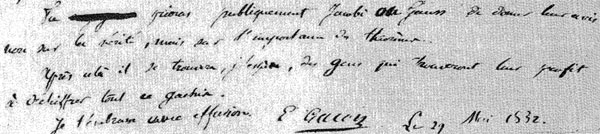Tu prieras publiquement Jacobi ou Gauss de donner leur avis non sur la verite, mais sur l’importance des theoremes.
Apres cela il se trouvera, j’espere, des gens qui trouvent leur profis a dechiffrer tout ce gachis.
Je t’embrasse avec effusion.
E. Galois, le 29 Mai 1832

A major result contained in this letter concerns the groups $L_2(p)=PSL_2(\mathbb{F}_p)$, that is the group of $2 \times 2$ matrices with determinant equal to one over the finite field $\mathbb{F}_p$ modulo its center. $L_2(p)$ is known to be simple whenever $p \geq 5$. Galois writes that $L_2(p)$ cannot have a non-trivial permutation representation on fewer than $p+1$ symbols whenever $p > 11$ and indicates the transitive permutation representation on exactly $p$ symbols in the three ‘exceptional’ cases $p=5,7,11$.

Let $\alpha = \begin{bmatrix} 1 & 1 \\ 0 & 1 \end{bmatrix}$ and consider for $p=5,7,11$ the involutions on $\mathbb{P}^1_{\mathbb{F}_p} = \mathbb{F}_p \cup { \infty }$ (on which $L_2(p)$ acts via Moebius transformations)

$\pi_5 = (0,\infty)(1,4)(2,3) \quad \pi_7=(0,\infty)(1,3)(2,6)(4,5) \quad \pi_{11}=(0,\infty)(1,6)(3,7)(9,10)(5,8)(4,2)$

(in fact, Galois uses the involution $~(0,\infty)(1,2)(3,6)(4,8)(5,10)(9,7)$ for $p=11$), then $L_2(p)$ leaves invariant the set consisting of the $p$ involutions $\Pi = { \alpha^{-i} \pi_p \alpha^i~:~1 \leq i \leq p }$. After mentioning these involutions Galois merely writes :

Ainsi pour le cas de $p=5,7,11$, l’equation modulaire s’abaisse au degre p.
En toute rigueur, cette reduction n’est pas possible dans les cas plus eleves.

Alternatively, one can deduce these permutation representation representations from group isomorphisms. As $L_2(5) \simeq A_5$, the alternating group on 5 symbols, $L_2(5)$ clearly acts transitively on 5 symbols.Similarly, for $p=7$ we have $L_2(7) \simeq L_3(2)$ and so the group acts as automorphisms on the projective plane over the field on two elements $\mathbb{P}^2_{\mathbb{F}_2}$ aka the Fano plane, as depicted on the left.

This finite projective plane has 7 points and 7 lines and $L_3(2)$ acts transitively on them.

For $p=11$ the geometrical object is a bit more involved. The set of non-squares in $\mathbb{F}_{11}$ is

${ 1,3,4,5,9 }$

and if we translate this set using the additive structure in $\mathbb{F}_{11}$ one obtains the following 11 five-element sets

${ 1,3,4,5,9 }, { 2,4,5,6,10 }, { 3,5,6,7,11 }, { 1,4,6,7,8 }, { 2,5,7,8,9 }, { 3,6,8,9,10 },$

${ 4,7,9,10,11 }, { 1,5,8,10,11 }, { 1,2,6,9,11 }, { 1,2,3,7,10 }, { 2,3,4,8,11 }$

and if we regard these sets as ‘lines’ we see that two distinct lines intersect in exactly 2 points and that any two distinct points lie on exactly two ‘lines’. That is, intersection sets up a bijection between the 55-element set of all pairs of distinct points and the 55-element set of all pairs of distinct ‘lines’. This is called the biplane geometry.

The subgroup of $S_{11}$ (acting on the eleven elements of $\mathbb{F}_{11}$) stabilizing this set of 11 5-element sets is precisely the group $L_2(11)$ giving the permutation representation on 11 objects.

An alternative statement of Galois’ result is that for $p > 11$ there is no subgroup of $L_2(p)$ complementary to the cyclic subgroup

$C_p = { \begin{bmatrix} 1 & x \\ 0 & 1 \end{bmatrix}~:~x \in \mathbb{F}_p }$

That is, there is no subgroup such that set-theoretically $L_2(p) = F \times C_p$ (note this is of courese not a group-product, all it says is that any element can be written as $g=f.c$ with $f \in F, c \in C_p$.

However, in the three exceptional cases we do have complementary subgroups. In fact, set-theoretically we have

$L_2(5) = A_4 \times C_5 \qquad L_2(7) = S_4 \times C_7 \qquad L_2(11) = A_5 \times C_{11}$

and it is a truly amazing fact that the three groups appearing are precisely the three Platonic groups!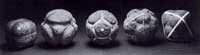Recall that here are 5 Platonic (or Scottish) solids coming in three sorts when it comes to rotation-automorphism groups : the tetrahedron (group $A_4$), the cube and octahedron (group $S_4$) and the dodecahedron and icosahedron (group $A_5$). The “4” in the cube are the four body diagonals and the “5” in the dodecahedron are the five inscribed cubes.

That is, our three ‘exceptional’ Galois-groups correspond to the three Platonic groups, which in turn correspond to the three exceptional Lie algebras $E_6,E_7,E_8$ via McKay correspondence (wrt. their 2-fold covers). Maybe I’ll detail this latter connection another time. It sure seems that surprises often come in triples…

Finally, it is well known that $L_2(5) \simeq A_5$ is the automorphism group of the icosahedron (or dodecahedron) and that $L_2(7)$ is the automorphism group of the Klein quartic.

So, one might ask : is there also a nice curve connected with the third group $L_2(11)$? Rumour has it that this is indeed the case and that the curve in question has genus 70… (to be continued).

Reference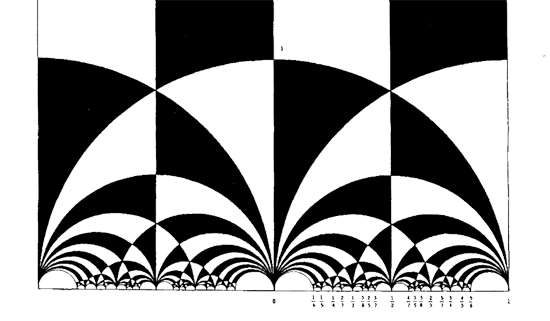The black&white psychedelic picture on the left of a tessellation of the hyperbolic upper-halfplane, was called the Dedekind tessellation in this post, following the reference given by John Stillwell in his excellent paper Modular Miracles, The American Mathematical Monthly, 108 (2001) 70-76.

But is this correct terminology? Nobody else uses it apparently. So, let’s try to track down the earliest depiction of this tessellation in the literature…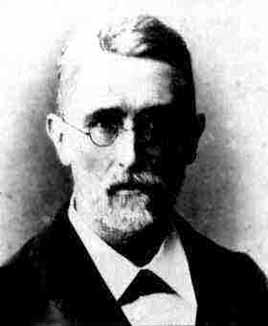Stillwell refers to Richard Dedekind‘s 1877 paper “Schreiben an Herrn Borchard uber die Theorie der elliptische Modulfunktionen”, which appeared beginning of september 1877 in Crelle’s journal (Journal fur die reine und angewandte Mathematik, Bd. 83, 265-292).

There are a few odd things about this paper. To start, it really is the transcript of a (lengthy) letter to Herrn Borchardt (at first, I misread the recipient as Herrn Borcherds which would be really weird…), written on June 12th 1877, just 2 and a half months before it appeared… Even today in the age of camera-ready-copy it would probably take longer.

There isn’t a single figure in the paper, but, it is almost impossible to follow Dedekind’s arguments without having a mental image of the tessellation. He gives a fundamental domain for the action of the modular group $\Gamma = PSL_2(\mathbb{Z})$ on the hyperbolic upper-half plane (a fact already known to Gauss) and goes on in section 3 to give a one-to-one mapping between this domain and the complex plane using what he calls the ‘valenz’ function $v$ (which is our modular function $j$, making an appearance in moonshine, and responsible for the black&white tessellation, the two colours corresponding to pre-images of the upper or lower half-planes).

Then there is this remarkable opening sentence.

Sie haben mich aufgefordert, eine etwas ausfuhrlichere Darstellung der Untersuchungen auszuarbeiten, von welchen ich, durch das Erscheinen der Abhandlung von Fuchs veranlasst, mir neulich erlaubt habe Ihnen eine kurze Ubersicht mitzuteilen; indem ich Ihrer Einladung hiermit Folge leiste, beschranke ich mich im wesentlichen auf den Teil dieser Untersuchungen, welcher mit der eben genannten Abhandlung zusammenhangt, und ich bitte Sie auch, die Ubergehung einiger Nebenpunkte entschuldigen zu wollen, da es mir im Augenblick an Zeit fehlt, alle Einzelheiten auszufuhren.

Well, just try to get a paper (let alone a letter) accepted by Crelle’s Journal with an opening line like : “I’ll restrict to just a few of the things I know, and even then, I cannot be bothered to fill in details as I don’t have the time to do so right now!” But somehow, Dedekind got away with it.

So, who was this guy Borchardt? How could this paper be published so swiftly? And, what might explain this extreme ‘je m’en fous’-opening ?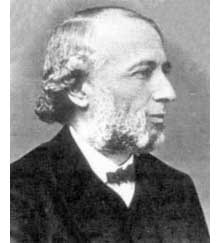Carl Borchardt was a Berlin mathematician whose main claim to fame seems to be that he succeeded Crelle in 1856 as main editor of the ‘Journal fur reine und…’ until 1880 (so in 1877 he was still in charge, explaining the swift publication). It seems that during this time the ‘Journal’ was often referred to as “Borchardt’s Journal” or in France as “Journal de M Borchardt”. After Borchardt’s death, the Journal für die Reine und Angewandte Mathematik again became known as Crelle’s Journal.

As to the opening sentence, I have a toy-theory of what was going on. In 1877 a bitter dispute was raging between Kronecker (an editor for the Journal and an important one as he was the one succeeding Borchardt when he died in 1880) and Cantor. Cantor had published most of his papers at Crelle and submitted his latest find : there is a one-to-one correspondence between points in the unit interval [0,1] and points of d-dimensional space! Kronecker did everything in his power to stop that paper to the extend that Cantor wanted to retract it and submit it elsewhere. Dedekind supported Cantor and convinced him not to retract the paper and used his influence to have the paper published in Crelle in 1878. Cantor greatly resented Kronecker’s opposition to his work and never submitted any further papers to Crelle’s Journal.

Clearly, Borchardt was involved in the dispute and it is plausible that he ‘invited’ Dedekind to submit a paper on his old results in the process. As a further peace offering, Dedekind included a few ‘nice’ words for Kronecker

Bei meiner Versuchen, tiefer in diese mir unentbehrliche Theorie einzudringen und mir einen einfachen Weg zu den ausgezeichnet schonen Resultaten von Kronecker zu bahnen, die leider noch immer so schwer zuganglich sind, enkannte ich sogleich…

Probably, Dedekind was referring to Kronecker’s relation between class groups of quadratic imaginary fields and the j-function, see the miracle of 163. As an added bonus, Dedekind was elected to the Berlin academy in 1880…

Anyhow, no visible sign of ‘Dedekind’s’ tessellation in the 1877 Dedekind paper, so, we have to look further. I’m fairly certain to have found the earliest depiction of the black&white tessellation (if you have better info, please drop a line). Here it is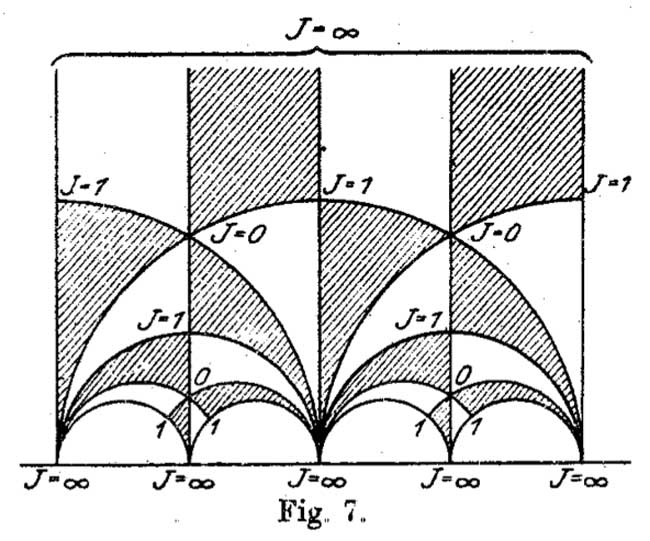It is figure 7 in Felix Klein‘s paper “Uber die Transformation der elliptischen Funktionen und die Auflosung der Gleichungen funften Grades” which appeared in may 1878 in the Mathematische Annalen (Bd. 14 1878/79). He even adds the j-values which make it clear why black triangles should be oriented counter-clockwise and white triangles clockwise. If Klein would still be around today, I’m certain he’d be a metapost-guru.

So, perhaps the tessellation should be called Klein’s tessellation??
Well, not quite. Here’s what Klein writes wrt. figure 7

Diese Figur nun – welche die eigentliche Grundlage fur das Nachfolgende abgibt – ist eben diejenige, von der Dedekind bei seiner Darstellung ausgeht. Er kommt zu ihr durch rein arithmetische Betrachtung.

Case closed : Klein clearly acknowledges that Dedekind did have this picture in mind when writing his 1877 paper!

But then, there are a few odd things about Klein’s paper too, and, I do have a toy-theory about this as well… (tbc)